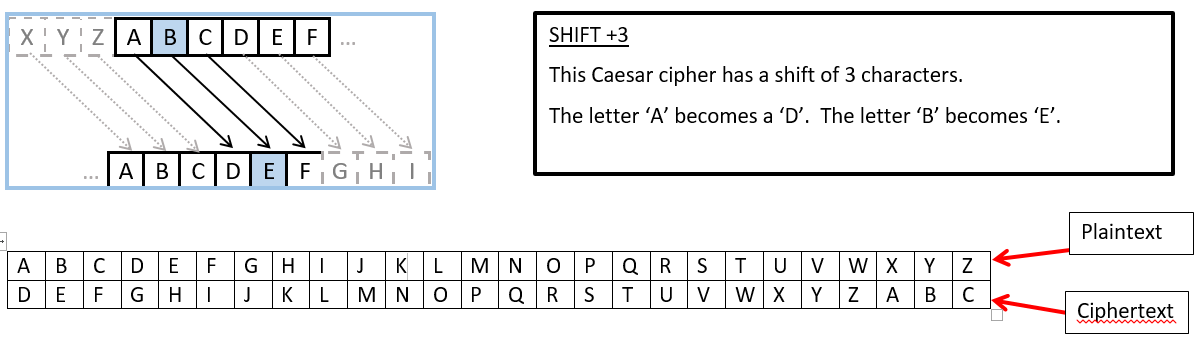Caesar Cipher - Part II¶

Total points possible Uses
10 LED display

Description¶

This challenge is about decrypting messages that have been encoded using the Caesar cipher. If you haven’t tried encrypting messages with the Caesar cipher then maybe you could attempt that first.

Using the Caesar cipher you can encrypt or decrypt all the letters in a message by shifting the alphabet a number of places. The figure below shows how to encrypt a message with a shift of 3 letters:Your goal is to turn your micro:bit into a machine that can decode messages that have been encrypted using the Caesar cipher. We call the encrypted message cipher text and the decrypted message plain text.

There is a trick you can use to decrypt, or shift the message. The trick relies on the fact that your micro:bit sees the letters of the alphabet as special numbers known as ascii values. You can translate a letter to an ascii number, and back again using the python functions ord() and chr().

Here is an example: Let’s say you have an encrypted message which has been shifted by 4 places. Try using this code to turn the character into a number and subtract 4:

ascii_char = ord(encrypted_char) - 4

In English this means: translate encrypted_char into an ascii number using the ord() function and subtract 4, the number of characters we want to shift.

But hold on, there is one more thing that we need to do. If you look at the picture above, you will see that we need to wrap around going from A back to Z. To do this we need to add 26 (the number of letters in the alphabet) if we have gone past A:

ascii_char = ord(plaintext_char) - 4
if ascii_char > ord('Z')
ascii_char = ascii_char + 26
encrypted_char = chr(ascii_char)

Try this out, experiment using the REPL.

Basic Challenge¶

Collect points for these stages: